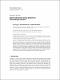## Joint Estimation Using Quadratic Estimating Function2011-7-12
##### Authors
Liang, Y.
Thavaneswaran, A.
Abraham, B.
##### Abstract
A class of martingale estimating functions is convenient and plays an important role for inference for nonlinear time series models. However, when the information about the first four conditional moments of the observed process becomes available, the quadratic estimating functions are more informative. In this paper, a general framework for joint estimation of conditional mean and variance parameters in time series models using quadratic estimating functions is developed. Superiority of the approach is demonstrated by comparing the information associated with the optimal quadratic estimating function with the information associated with other estimating functions. The method is used to study the optimal quadratic estimating functions of the parameters of autoregressive conditional duration (ACD) models, random coefficient autoregressive (RCA) models, doubly stochastic models and regression models with ARCH errors. Closed-form expressions for the information gain are also discussed in some detail.
##### Citation
Y. Liang, A. Thavaneswaran, and B. Abraham, “Joint Estimation Using Quadratic Estimating Function,” Journal of Probability and Statistics, vol. 2011, Article ID 372512, 14 pages, 2011. doi:10.1155/2011/372512